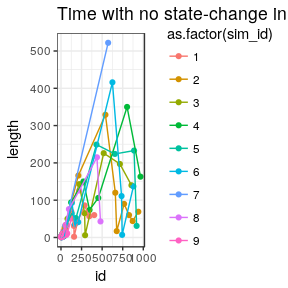# Studying the behaviour of Kendall random walks

We will approximate the distribution of moments when the random walk changes state through simulations.

First, we simulate many paths of Kendall random walk with normal step distribution.

library(kendallRandomWalks)
library(dplyr)
library(ggplot2)
set.seed(17)
walks <- simulate_kendall_rw(1000, 1000, rnorm, 0.5, T)

Example trajectory

plot(walks, max_id = 1)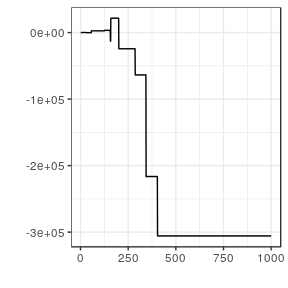Number of unique states

ggplot(summarise_kendall_rw(walks, n_distinct), aes(x = aggregated)) +
# geom_histogram() +
theme_bw() +
geom_density()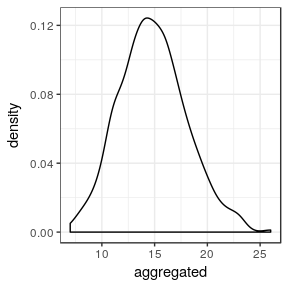Jumps

diffs <- mutate_kendall_rw(walks, function(x) x - lag(x))
diffs
#> # A tibble: 1,000,000 x 2
#> # Groups:   sim_id [1,000]
#>    sim_id     sim
#>     <int>   <dbl>
#>  1      1  NA
#>  2      1  -1.02
#>  3      1   0.
#>  4      1  -0.478
#>  5      1   3.88
#>  6      1  -5.04
#>  7      1  26.0
#>  8      1   0.
#>  9      1   0.
#> 10      1   0.
#> # ... with 999,990 more rows
diffs2 <- mutate_kendall_rw(walks, function(x) x - lag(x), F)
plot(diffs2, max_id = 10)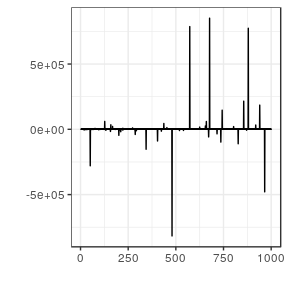Time with no change of state

diffs3 <- mutate_kendall_rw(diffs2, function(x) as.numeric(x != 0), F)
diffs <- diffs3\$simulation
diffs <- group_by(diffs, sim_id) %>%
mutate(id = 1:n())
lengths <- diffs %>%
filter(sim != 0) %>%
mutate(previous = ifelse(is.na(lag(id)), 0, lag(id))) %>%
mutate(length = id - previous)
ggplot(subset(lengths, sim_id < 5),
aes(x = length, fill = as.factor(sim_id), group = as.factor(sim_id))) +
geom_density() +
theme_bw() +
ggtitle("Distribution of time with no state-change (by simulation)")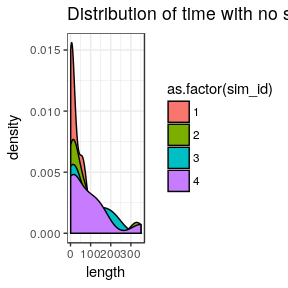ggplot(lengths, aes(x = length)) +
geom_density() +
theme_bw() +
ggtitle("Distribution of time with no state-change (aggregated)")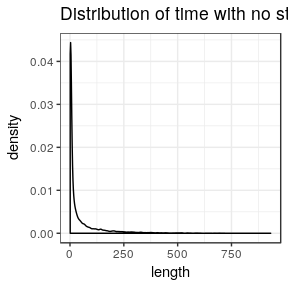ggplot(subset(lengths, sim_id < 10), aes(x = id, y = length, color = as.factor(sim_id))) +
geom_point() +
theme_bw() +
geom_line() +
ggtitle("Time with no state-change in time")